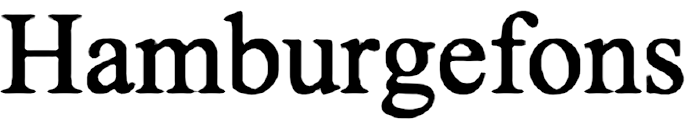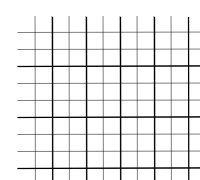# Essential Math WeblogThoughts on math for games

## 3/19/2015

### Rendering Signed Distance Fields, Part 2

Filed under: Mathematical,Tutorial — Jim @ 9:32 pm

So in Part 1 of this series, I talked about how to create a shader to render a distance field texture that only has uniform scale, rotation or translation applied — no shear or perspective transformations. So now one might expect (as I promised) that I’d talk about how to create a general shader for distance fields. After looking at what I wrote, however, I realized that a) I need to explain why you might want to use a signed distance field texture and b) to explain the rest I need to bring everyone up to speed on multivariable derivatives — namely gradients and the Jacobian. So I’ll cover those in this post, and finish up with the full answer next time. That said, I did fix the bug in Skia, so if you know all this or are impatient, you can skip ahead and look at the source code.

Why Use Distance Fields?

The motivation for games comes primarily from Chris Green’s paper “Improved Alpha-Tested Magnification for Vector Textures and Special Effects”. This in turn comes from a paper by Qin et al., “Real-time texturemapped vector glyphs.” What we’re trying to represent is the outline of a shape. A normal alpha-blended or alpha-tested bitmap decal image has aliasing on the edges once you transform it beyond its original size and shape. Distance field images can be scaled and generally transformed while maintaining reasonably sharp edges. And since you are representing the distance to a shape, rather than the shape itself, you can apply special effects to this. For example, you could render the shape with a stroked line, rather than filled. Both Green and Qin have examples of this.

That said, I’m not going to say there’s no distortion, because you can only scale a distance field image down so far before it starts looking like a blurry mess, and you can only scale it up so far before you start noticing artifacts. First, any points of fine detail like sharp points start looking rather rounded. Secondly, some edges start looking like they’ve been chewed on (thanks to Behdad Esfahbod for pointing this particular case out to me):The reason for this is that a distance field, like any other texture, is a sampling. And like any sampling, it can only be used to reconstruct the original function up to a point (look up Nyquist frequency). To get a good reconstruction at a higher resolution, you need a finer sampling.

But with all of that, you do get a fair amount of range out of them. Because of this, they’re also useful for representing text — you can store one scale of glyphs in a texture atlas, and get a large range of font sizes out of them. Skia can use distance fields to represent both glyphs and small paths.

So hopefully that provides enough justification for all of this.

A Brief Review of Multivariable Calculus

So most people who’ve done calculus have seen single variable functions, where you input one value and get as output one value — e.g, $f(x) = x^2 + 2x + 1$. The derivative of such a function also produces one value, or in this case $f'(x) = 2x + 2$.

But what if we have a function that takes two values and outputs one? An example of this might be a height field, where $(x, y)$ represents a position and $f(x,y)$ is the height at that point. Or, say, a distance field. The derivative of such a function is called the gradient. It’s represented as $\nabla f$, and is a vector pointing in the direction of greatest change at the point $(x, y)$, and the length of that vector represents the rate of change. If you think of a height field, the gradient vector for a position points in the direction of the steepest slope at that position.

To compute the gradient for these 2D functions, you take the partial derivatives of the function with respect to x and y and output as a vector. This means you treat x (or y) as the only variable and everything else as a constant. For example, the implicit function for a circle is $f(x, y) = x^2 + y^2 – r^2$. The gradient is then $\nabla f(x, y) = (\partial f/\partial x, \partial f/\partial y) = (2x, 2y)$.

For a function that outputs a vector (also called a vector-valued function), the equivalent derivative is the Jacobian matrix. So for example, suppose we have a transformation $T$ that takes a position $(x,y)$ in screen space and outputs a position $(u, v)$ in texel coordinates. The Jacobian $\mathbf{J}$ is just a matrix of all the possible partial derivatives, or
$\mathbf{J} = \left[ \begin{array}{cc} \partial u / \partial x & \partial u / \partial y \\ \partial v / \partial x & \partial v / \partial y \end{array} \right]$

In the fragment shader, we can approximate the gradient and Jacobian for various entities relative to the fragment’s position using the dFdx and dFdy functions (see Chapter 9 of Essential Math for Games to see how these differentials can be calculated during rasterization). So the approximate gradient of the distance would be
 vec2 gradient = vec2(dFdx(distance), dFdy(distance)); 

And the approximate Jacobian of the texel coordinates (broken into two column vectors) is
 in vec2 uv; // [0..width][0..height] ... vec2 Jdx = dFdx(uv); vec2 Jdy = dFdy(uv); 
Let’s look at this last in more detail. First, to be clear, when I say texel coordinates I mean non-normalized texture coordinates — they range from 0 to the texture size, not from 0 to 1. Suppose we take our glyph in uv texel space and apply the following to transform it to xy pixel space:
$\mathbf{M} = \left[ \begin{array}{ccc} 2 & 0 & 0 \\ 0 & 3 & 0 \\ 0 & 0 & 1 \end{array} \right]$
The difference in texel and pixel space would look something like this:Here the thin lines represent the pixel grid, and the thick lines represent the texel grid. dFdx() computes the change in a value given a step in the x direction. So looking at the grid, if we step 1 pixel in x, we step 1/2 a texel in u, and 0 texels in v. Similarly for dFdy(), if we step 1 pixel in y, we step 1/3 a texel in v, and 0 texels in u. So the Jacobian for this example is
$\mathbf{J} = \left[ \begin{array}{cc} \partial u / \partial x & \partial u / \partial y \\ \partial v / \partial x & \partial v / \partial y \end{array} \right] = \left[ \begin{array}{cc} 1/2 & 0 \\ 0 & 1/3 \end{array} \right]$

which is just the upper 2×2 of the 2D inverse transformation matrix. More generally, the Jacobian can give us a linear approximation to the inverse transformation for a fragment. This is particularly useful for perspective transformations, where the transformation is non-linear in pixel space, so we can’t just pass in a matrix as a uniform or constant.

And with that, I think we’re ready to discuss the general distance field shader.